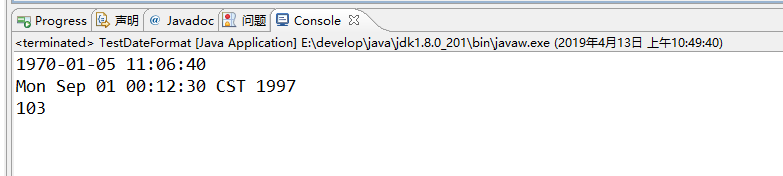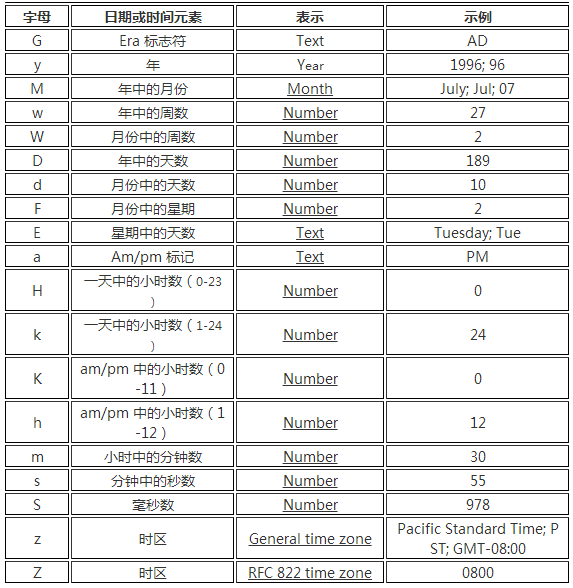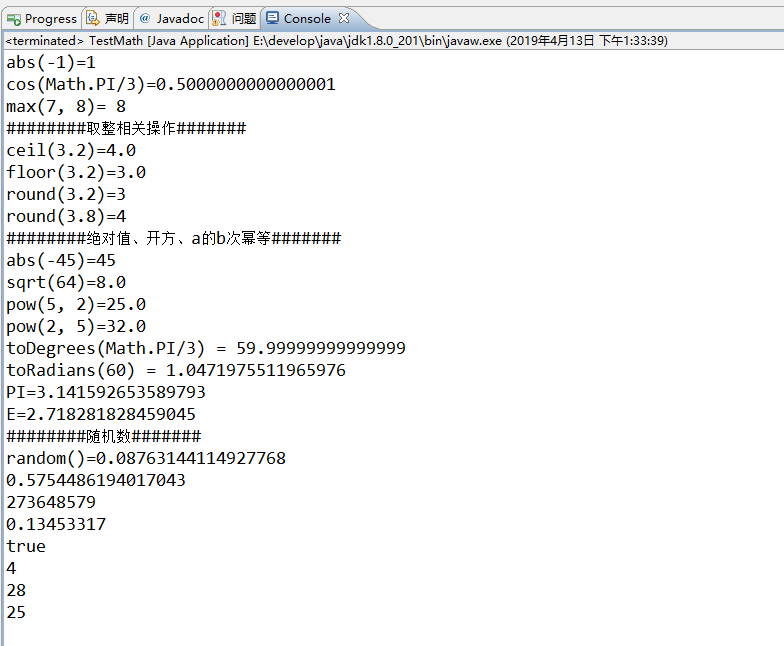JAVA

# java复习笔记（四）

java复习笔记 四

### 笔记

#### StringBuilder和StringBuffer可变字符序列

StringBuffer JDK1.0版本提供的类，线程安全，做线程同步检查， 效率较低。

StringBuilder JDK1.5版本提供的类，线程不安全，不做线程同步检查，因此效率较高。一般用StringBuilder的比较多

StringBuilder常用的方法：

1. 重载的append(…)为该StringBuilder对象追加单个字符，并且返回自身对象
``````sb.append(‘a’);//追加单个字符
sb.append(" I am coming!");//追加字符串
``````
2. delete(int start,int end)方法，可以删除从start开始，到end-1为止的一段字符序列，并且返回自身对象

3. deleteCharAt(int index);方法，删除在指定位置上的字符，并且返回自身对象

4. toString()方法，返回序列中数据的字符串表示形式

``````sb.toString()
``````
5. 与String类含义类似的方法
``````public int indexOf(String str)//返回从头开始查找，指定字符串第一次出现的索引值。若没有则返回-1
public int indexOf(String str,int fromIndex)
public String substring(int start)//提取从指定索引开始到结尾的字符串
public String substring(int start,int end)//提取指定索引区间之间的字符，不包括后面end的索引！！
public int length()
char charAt(int index)//提取指定索引的字符
``````

#### 不可变与可变字符序列在使用过程中的问题

String经过初始化后，就不会改变内容了。对字符串的操作实际上是对其副本的操作。String s = “a”;s = s + “b”;实际上原来的“a”字符串对象已经丢弃了，产生了新的字符串对象“ab”,多次执行会导致大量副本留在内存中，影响性能。而使用StringBuilder和StringBuffer就不会产生这个问题，因为他们是对原字符串本身进行操作的。

#### DateFormat抽象类和SimpleDateFormat实现类

DateFormat把时间对象转化成指定格式的字符串。反之，把指定格式的字符串转化成时间对象。 DateFormat是一个抽象类，一般使用它的子类SimpleDateFormat类来实现。

使用示例如下：

``````package cn.xgblack.studyClass;

import java.text.DateFormat;
import java.text.ParseException;
import java.text.SimpleDateFormat;
import java.util.Date;

/**
* 测试时间对象和字符串之间的相互转换
* DateFormat抽象类和SimpleDateFormat实现类的使用
* @author xg
* @time 2019年4月13日
*/
public class TestDateFormat{

public static void main(String[] args) throws ParseException{

//把时间对象按照“格式字符串指定格式”转成相应的字符串
DateFormat df = new SimpleDateFormat("yyyy-MM-dd hh:mm:ss");
String str = df.format(new Date(400000000));
System.out.println(str);

//把字符串按照“格式字符串指定格式”转成相应的时间对象
DateFormat df2 = new SimpleDateFormat("yyyy年MM月dd日 hh时mm分ss秒");
Date date = df2.parse("1997年09月01日 12时12分30秒");
System.out.println(date);

//测试其他格式字符
DateFormat df3 = new SimpleDateFormat("D"); //D 对象是所处年份的第几天
String str2 = df3.format(new Date());
System.out.println(str2);
}

}

``````#### Calendar日历类

Calendar类是抽象类，提供日起计算相关功能。年月日时分秒的展示和计算。GregorianCalendar是Calendar的具体子类。

#### Math类

``````package cn.xgblack.studyClass;

import java.util.Random;

public class TestMath {
public static void main(String[] args) {
System.out.println("abs(-1)=" + Math.abs(-1));//绝对值
System.out.println("cos(Math.PI/3)=" + Math.cos(Math.PI/3));//三角函数：acos,asin,atan,cos,sin,tan  角度为弧度值
System.out.println("max(7, 8)= " + Math.max(7, 8));//最大值。      min（）最小值

//取整相关操作
System.out.println("########取整相关操作#######");
System.out.println("ceil(3.2)=" + Math.ceil(3.2));//大于3.2的最小整数，即去掉小数进1
System.out.println("floor(3.2)=" + Math.floor(3.2));//小于3.2的最大整数，即舍去小数部分
System.out.println("round(3.2)=" + Math.round(3.2));//四舍五入
System.out.println("round(3.8)=" + Math.round(3.8));//四舍五入
//绝对值、开方、a的b次幂等操作
System.out.println("########绝对值、开方、a的b次幂等#######");
System.out.println("abs(-45)=" + Math.abs(-45));//绝对值
System.out.println("sqrt(64)=" + Math.sqrt(64));//平方根
System.out.println("pow(5, 2)=" + Math.pow(5, 2));//5的2次方
System.out.println("pow(2, 5)=" + Math.pow(2, 5));//2的5次方

System.out.println("toDegrees(Math.PI/3) = " + Math.toDegrees(Math.PI/3));//弧度转换为角度

//Math类中常用的常量
System.out.println("PI=" + Math.PI);
System.out.println("E=" + Math.E);

//随机数
System.out.println("########随机数#######");
System.out.println("random()=" + Math.random());// [0,1)

Random rand = new Random();
//随机生成[0,1)之间的double类型的数据
System.out.println(rand.nextDouble());
//随机生成int类型允许范围之内的整型数据
System.out.println(rand.nextInt());
//随机生成[0,1)之间的float类型的数据
System.out.println(rand.nextFloat());
//随机生成false或者true
System.out.println(rand.nextBoolean());
//随机生成[0,10)之间的int类型的数据
System.out.println(rand.nextInt(10));
//随机生成[20,30)之间的int类型的数据
System.out.println(20 + rand.nextInt(10));
//随机生成[20,30)之间的int类型的数据（此种方法计算较为复杂）
System.out.println(20 + (int) (rand.nextDouble() * 10));
}
}
``````#### File类

java.io.File类：代表文件和目录。 在开发中，读取文件、生成文件、删除文件、修改文件的属性时经常会用到File类。

``````File f = new File("d:/b.txt");
System.out.println("File是否存在："+f.exists());
System.out.println("File是否是目录："+f.isDirectory());
System.out.println("File是否是文件："+f.isFile());
System.out.println("File最后修改时间："+new Date(f.lastModified()));
System.out.println("File的大小："+f.length());
System.out.println("File的文件名："+f.getName());
System.out.println("File的目录路径："+f.getPath());//相对路径
System.out.println("File的目录路径："+f.getAbsolutePath());//绝对路径
``````

mkdir与mkdirs

``````File f2 = new File("d:/电影/华语/大陆");
boolean flag = f2.mkdir(); //目录结构中有一个不存在，则不会创建整个目录树
System.out.println(flag);//创建失败
boolean flag = f2.mkdirs(); //创建整个目录树
System.out.println(flag);//创建成功
``````
0 条回应